Published

# Asset Management in Remote Location

Asset Management and theft detection in remote location using edge impulse and blues wireless

IntermediateFull instructions provided3 hours250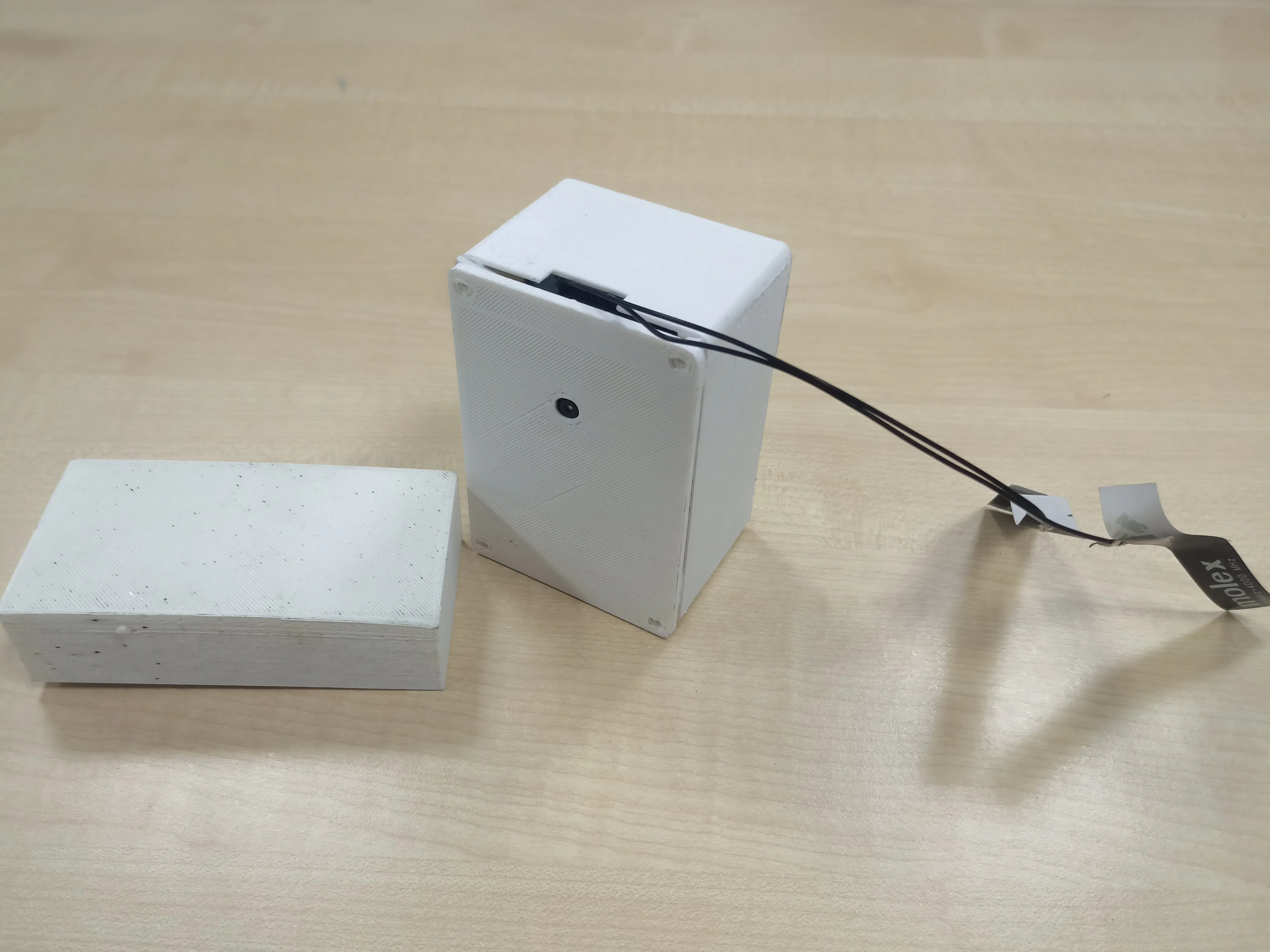## Things used in this project

### Hardware components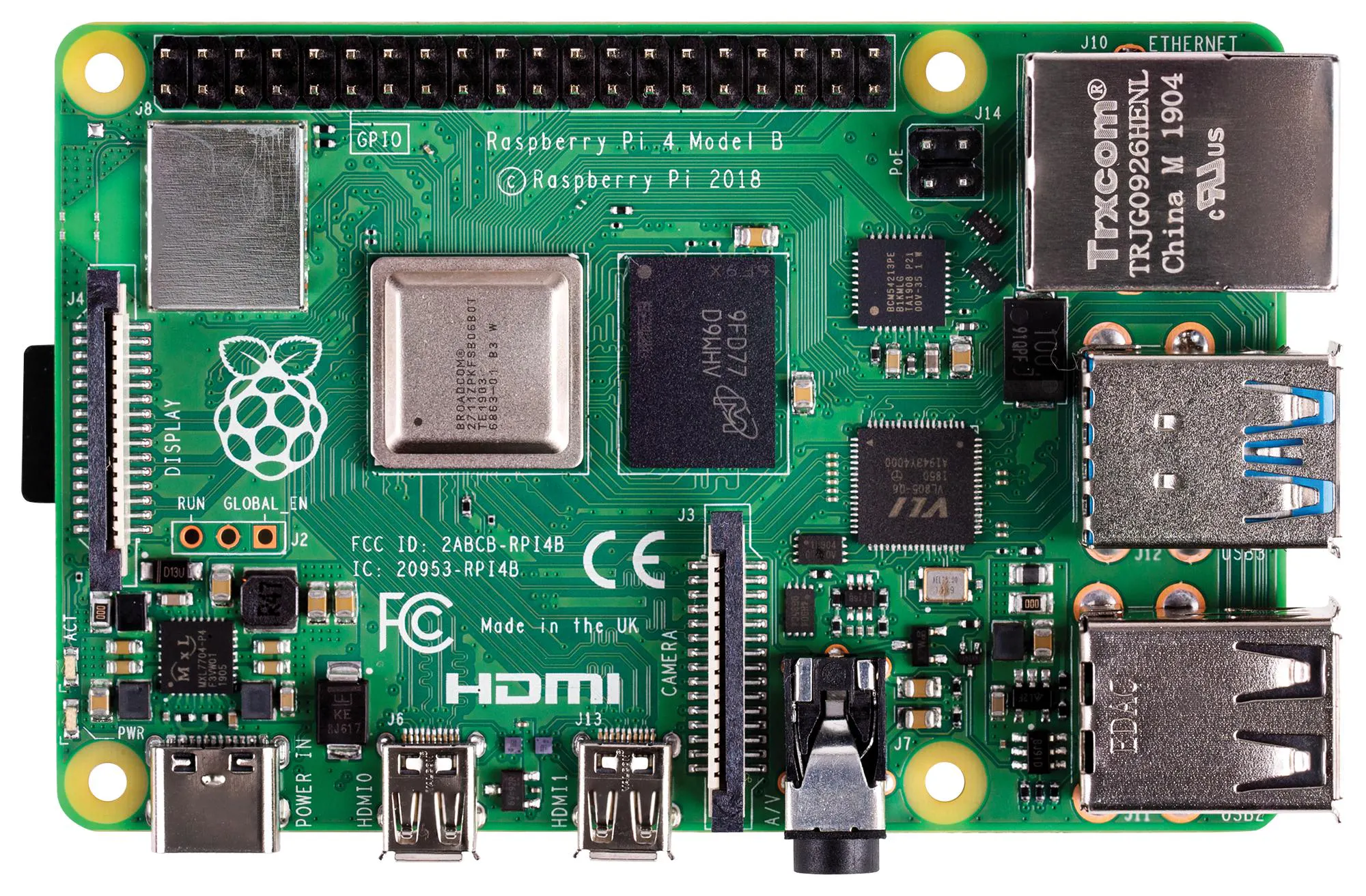Raspberry Pi 4 Model B
×1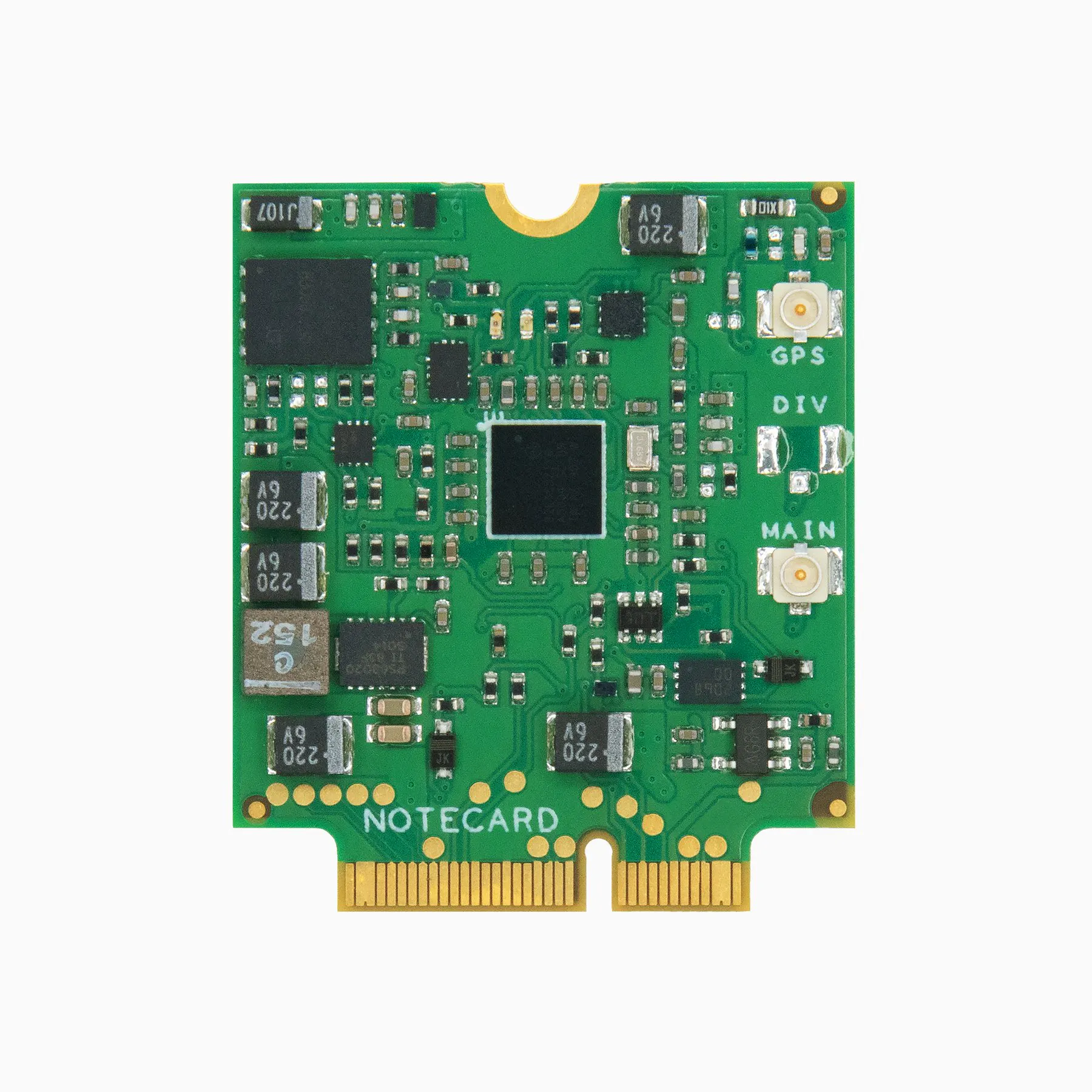Blues Notecard (Cellular)
×1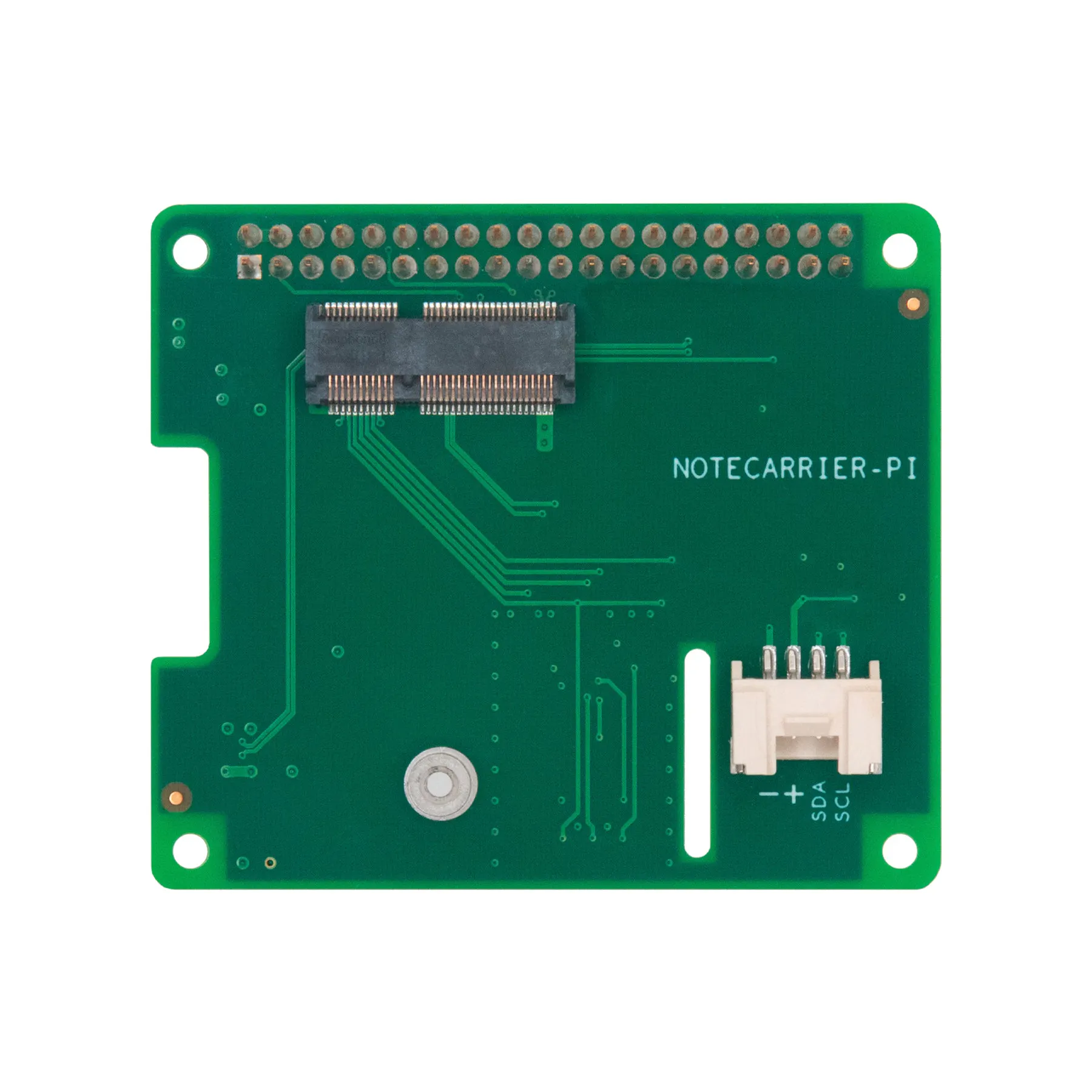Blues Notecarrier-Pi
×1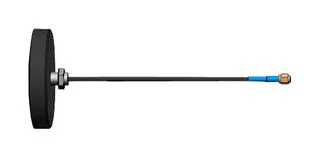Antenna, Cellular / LTE
×1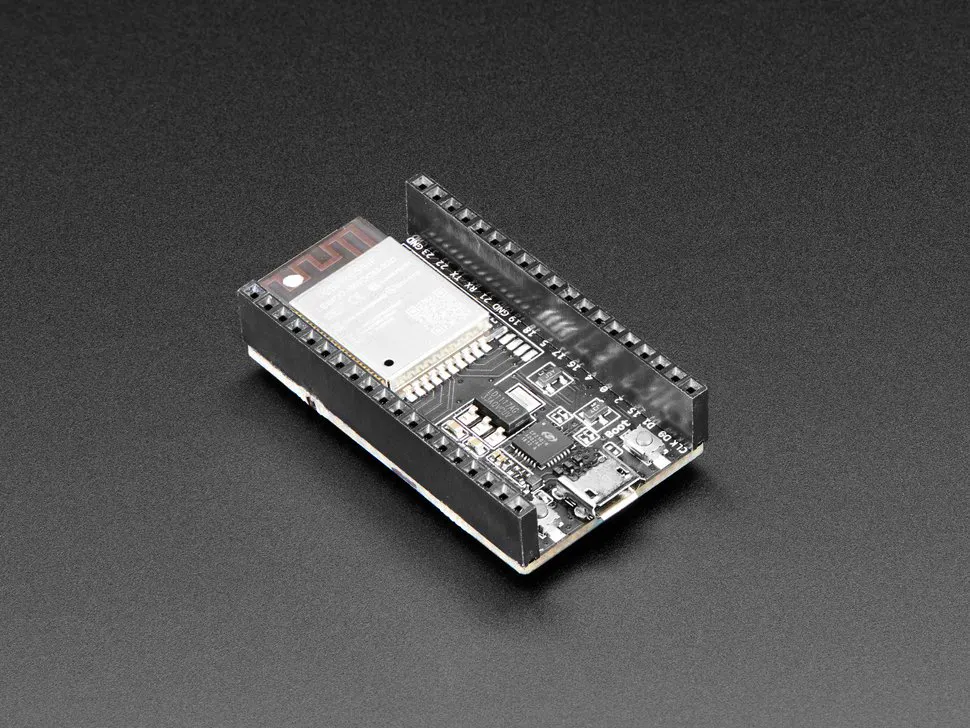Espressif ESP32 Development Board - Developer Edition
×1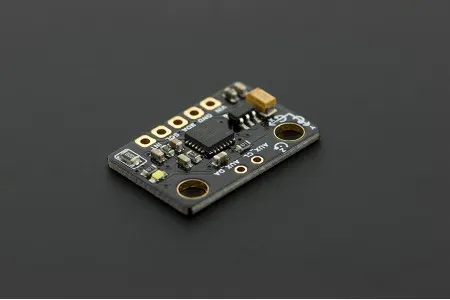DFRobot 6 DOF Sensor - MPU6050
×1

### Software apps and online servicesArduino IDEEdge Impulse Studio

### Hand tools and fabrication machines3D Printer (generic)

## Custom parts and enclosures

### RPI Case

Enclosure for RPI and Notecarrier

## Schematics

### Schematic _ Arduino

Arduino MPU4050 schematic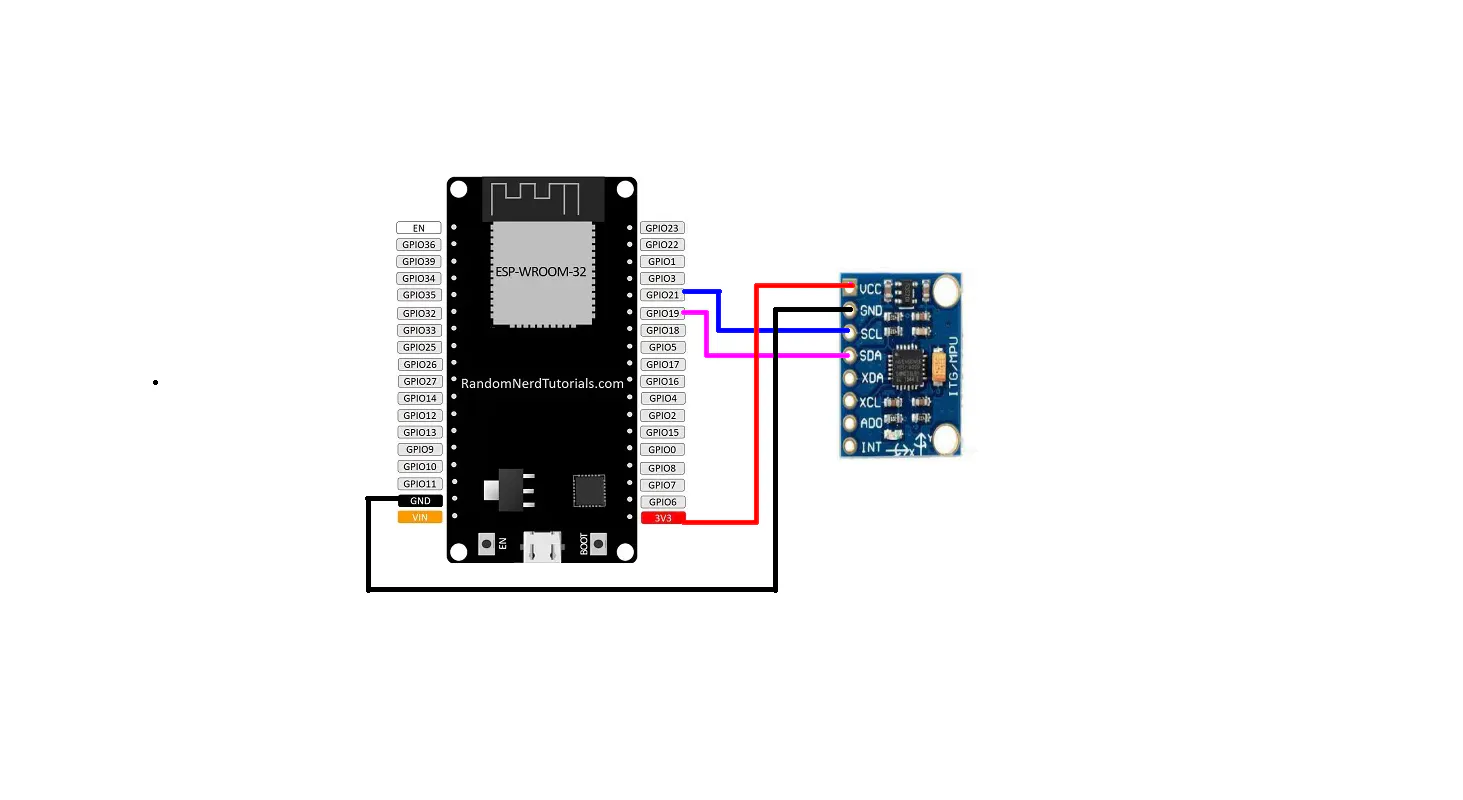## Code

### Arduino Code

Arduino
```#include <Adafruit_MPU6050.h>
#include <Wire.h>
#include <Asset_Management_inferencing.h>
#include <Arduino.h>
#include <ArduinoBLE.h>

uint8_t manufacturerData={0x01,0x02,0x03,0x04,0x05,0x07};
uint8_t current_state = 1;
uint8_t prev_state = 0;
bool dataset = 1;

#define FREQUENCY_HZ        60
#define INTERVAL_MS         (1000 / (FREQUENCY_HZ + 1))
#define RED 16
#define GREEN 17
#define BLUE 18

float features[EI_CLASSIFIER_DSP_INPUT_FRAME_SIZE];
size_t feature_ix = 0;

static unsigned long last_interval_ms = 0;

void setup() {
Serial.begin(115200);

if (!BLE.begin())
{
Serial.println("starting BLE failed!");
while (1)
;
}

BLE.setLocalName("Asset1");

Wire.setPins(19,21);
if (!mpu.begin()) {
Serial.println("Failed to find MPU6050 chip");
while (1) {
delay(10);
}
}
Serial.println("MPU6050 Found!");

mpu.setAccelerometerRange(MPU6050_RANGE_8_G);
Serial.print("Accelerometer range set to: ");
switch (mpu.getAccelerometerRange()) {
case MPU6050_RANGE_2_G:
Serial.println("+-2G");
break;
case MPU6050_RANGE_4_G:
Serial.println("+-4G");
break;
case MPU6050_RANGE_8_G:
Serial.println("+-8G");
break;
case MPU6050_RANGE_16_G:
Serial.println("+-16G");
break;
}
mpu.setGyroRange(MPU6050_RANGE_500_DEG);
Serial.print("Gyro range set to: ");
switch (mpu.getGyroRange()) {
case MPU6050_RANGE_250_DEG:
Serial.println("+- 250 deg/s");
break;
case MPU6050_RANGE_500_DEG:
Serial.println("+- 500 deg/s");
break;
case MPU6050_RANGE_1000_DEG:
Serial.println("+- 1000 deg/s");
break;
case MPU6050_RANGE_2000_DEG:
Serial.println("+- 2000 deg/s");
break;
}

mpu.setFilterBandwidth(MPU6050_BAND_21_HZ);
Serial.print("Filter bandwidth set to: ");
switch (mpu.getFilterBandwidth()) {
case MPU6050_BAND_260_HZ:
Serial.println("260 Hz");
break;
case MPU6050_BAND_184_HZ:
Serial.println("184 Hz");
break;
case MPU6050_BAND_94_HZ:
Serial.println("94 Hz");
break;
case MPU6050_BAND_44_HZ:
Serial.println("44 Hz");
break;
case MPU6050_BAND_21_HZ:
Serial.println("21 Hz");
break;
case MPU6050_BAND_10_HZ:
Serial.println("10 Hz");
break;
case MPU6050_BAND_5_HZ:
Serial.println("5 Hz");
break;
}

Serial.println("");
delay(100);

Serial.print("Features: ");
Serial.println(EI_CLASSIFIER_DSP_INPUT_FRAME_SIZE);
Serial.print("Label count: ");
Serial.println(EI_CLASSIFIER_LABEL_COUNT);

}

void loop() {
sensors_event_t a, g, temp;

if (millis() > last_interval_ms + INTERVAL_MS) {
last_interval_ms = millis();

mpu.getEvent(&a, &g, &temp);

features[feature_ix++] = a.acceleration.x;
features[feature_ix++] = a.acceleration.y;
features[feature_ix++] = a.acceleration.z;

if (feature_ix == EI_CLASSIFIER_DSP_INPUT_FRAME_SIZE) {
//Serial.println("Running the inference...");
signal_t signal;
ei_impulse_result_t result;
int err = numpy::signal_from_buffer(features, EI_CLASSIFIER_DSP_INPUT_FRAME_SIZE, &signal);
if (err != 0) {
ei_printf("Failed to create signal from buffer (%d)\n", err);
return;
}

EI_IMPULSE_ERROR res = run_classifier(&signal, &result, true);

if (res != 0) return;

/*ei_printf("Predictions ");
ei_printf("(DSP: %d ms., Classification: %d ms.)",
result.timing.dsp, result.timing.classification);
ei_printf(": \n");*/
Serial.println("Predictions");
Serial.printf("(DSP: %d ms., Classification: %d ms.)",
result.timing.dsp, result.timing.classification);
for (size_t ix = 0; ix < EI_CLASSIFIER_LABEL_COUNT; ix++) {
//ei_printf("    %s: %.5f\n", result.classification[ix].label, result.classification[ix].value);
Serial.printf("%s: %.5f\n", result.classification[ix].label, result.classification[ix].value);
if (result.classification[ix].value > 0.6) {
if (result.classification[ix].label == "Falling")
{
Serial.println("Asset is Falling");
current_state = 2;

} else if (result.classification[ix].label == "Movement")
{
Serial.println("Asset is Moving");
current_state = 3;
} else if (result.classification[ix].label == "Tampering")
{
Serial.println("Asset is Tampering");
current_state =4;
} else
{
Serial.println("Asset is in IDLE");
current_state = 5;
}
}
}
feature_ix = 0;
}

}

if (prev_state != current_state){
prev_state = current_state;
dataset = 0;
manufacturerData=current_state;

}

}

void ei_printf(const char *format, ...) {
static char print_buf = { 0 };

va_list args;
va_start(args, format);
int r = vsnprintf(print_buf, sizeof(print_buf), format, args);
va_end(args);

if (r > 0) {
Serial.write(print_buf);
}
}
```

### Python Code in RPi

Python
Scan the beacon and update data to cloud
```from bluepy.btle import Scanner, DefaultDelegate
import json
import notecard
import notecard_pseudo_sensor
from periphery import I2C
import time

current_state = "1"
prev_state = "0"
productUID = "com.gmail.thareeq123:asset_management"
port = I2C("/dev/i2c-1")
card = notecard.OpenI2C(port, 0, 0)
req = {"req": "hub.set"}
req["product"] = productUID
req["mode"] = "continuous"
print(json.dumps(req))
rsp = card.Transaction(req)
print(rsp)

class ScanDelegate(DefaultDelegate):

def __init__(self):
DefaultDelegate.__init__(self)

def handleDiscovery(self, dev, isNewDev, isNewData):
#if isNewDev:
#elif isNewData:
for (adtype, desc, value) in dev.getScanData():
if(desc=="Manufacturer"):
data = value
if(desc=="Complete Local Name" and value=="Asset1"):
print("  %s = %s" % (desc, value))
print(f"MAnufactureer data {data} and {type(data)}")
global current_state
if data=="5":
print("Device is IDLE state")
current_state = "IDLE"
elif data=="3":
print("Device is Moving")
current_state = "Moving"
elif data=="2":
print("Device is Falling")
current_state = "Falling"
elif data=="4":
print("Device is Tampering")
current_state = "Tampered"

scanner = Scanner().withDelegate(ScanDelegate())
def scanning_func():
while True:
devices = scanner.scan()

x.start()

while True:
if prev_state != current_state:
prev_state=current_state
req["file"] = "sensors.qo"
req["sync"] = True
req["body"] = { "Package": "Asset1", "State": current_state }
rsp = card.Transaction(req)
print(rsp)
print("Data send to cloud")
```

## Credits

### Thareeq Anwar k

5 projects • 5 followers